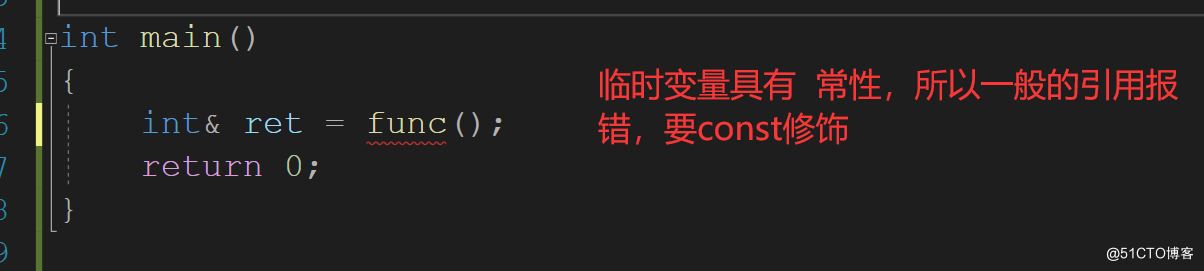# C++ 引用與內聯函數詳情

## 引用初階

### 什麼是引用

```#include <stdio.h>
int main()
{
int a = 10;
int& b = a;       //  b 是 a的一個別名
printf("%p\n", &a);
printf("%p\n", &b);
return 0;
}```### 為何要有引用

```#include <stdio.h>
void swap(int& a, int& b)
{
int c = a;
a = b;
b = c;
}
int main()
{
int a = 10;
int b = 20;
printf("交換前  a = %d b = %d\n", a, b);
swap(a, b);
printf("交換後  a = %d b = %d\n", a, b);
return 0;
}```### 引用指向同一塊空間

```#include <iostream>
using namespace std;
int main()
{
int a = 10;
int& b = a;  //b 是  a  的別名
int& c = b;  //還可以對c取別名
return 0;
}``````int main()
{
int a = 10;
int& b = a;  //b 是  a  的別名
int& c = b;  //還可以對c取別名
c = 20;
cout << "a: " << a << endl;
cout << "b: " << a << endl;
cout << "c: " << a << endl;
return 0;
}```### 引用的特性

#### 定義時必須初識化

```#include <iostream>
using namespace std;
int main()
{
int a = 10;
int& b;
return 0;
}```#### 引用一旦引用瞭一個實例,不能在再引用其他的實例

```int main()
{
int a = 10;
int& b = a;
int c = 20;
// 這個一定是賦值
b = c;
cout << "a: " << a << endl;
return 0;
}
```## 引用進階

### 常引用

```const int a = 10;
```

```int main()
{
const int a = 10;
int& b = a;        //報錯
const int& c = a;  // 不報錯
return 0;
}```#### 權限

```int main()
{
int a = 10;
const int& b = a;
return 0;
}
``````int main()
{
const int& a = 10;
return 0;
}```#### 臨時變量具有常屬性

```int main()
{
double d = 1.2;
const int& a = d;       //需要用const修飾
cout << a << endl;
return 0;
}``````int main()
{
double d = 1.2;
const int& a = d;
cout << "&a :" <<&a << endl;
cout << "&d :" <<&d << endl;
return 0;
}```### 引用的場景

• 做參數
• 做返回值

#### 做參數

```#include <stdio.h>
void swap(int& a, int& b)
{
int c = a;
a = b;
b = c;
}
int main()
{
int a = 10;
int b = 20;
printf("交換前  a = %d b = %d\n", a, b);
swap(a, b);
printf("交換後  a = %d b = %d\n", a, b);
return 0;
}``````void func(int& a)
{
}
int main()
{
int a = 10;
double b = 10.2;

func(a);
func(10);
func(b);
return 0;
}```#### 返回值

```int func()
{
int n = 1;
n++;
return n;
}

int main()
{
int ret = func();
return 0;
}``````int main()
{
int& ret = func();
return 0;
}
```#### 引用做返回值

```int& func()
{
static int n = 1;
n++;
return n;
}
int main()
{
int ret = func();
cout << ret << endl;
return 0;
}``````int& func()
{
static int n = 1;
n++;
cout << &n << endl;
return n;
}

int main()
{
int& ret = func();     //  註意 是 int&
cout << &ret << endl;
return 0;
}``````int& func()
{
int n = 1;
n++;
return n;
}

int main()
{
int& ret = func();
printf("這是一條華麗的分割線\n");
cout << ret << endl;
cout << ret << endl;
return 0;
}```### 引用不會開辟空間

```typedef struct A
{
int arr;
} A;

void func1(A a)
{

}

void func2(A& a)
{

}

void TestRefAndValue()
{
A a;

// 以值作為函數參數
size_t begin1 = clock();
for (size_t i = 0; i < 100000; ++i)
func1(a);
size_t end1 = clock();

// 以引用作為函數參數
size_t begin2 = clock();
for (size_t i = 0; i < 100000; ++i)
func2(a);
size_t end2 = clock();

// 分別計算兩個函數運行結束後的時間
cout << "func1(A)-time:" << end1 - begin1 << endl;
cout << "func2(A&)-time:" << end2 - begin2 << endl;
}

int main()
{
TestRefAndValue();
return 0;
}
```### 引用和指針比較

• 引用不會開辟空間,但是指針會開辟相應的空間.

```int main()
{
int a = 10;
//語法沒有開辟空間  底層開辟瞭
int& b = a;
b = 20;

//語法開辟空間  底層也開辟瞭
int* pa = &a;
*pa = 20;
return 0;```• 引用在定義時必須初始化，指針沒有要求
• 引用在初始化時引用一個實體後，就不能再引用其他實體，而指針可以在任何時候指向任何一個同類型實體
• 沒有NULL引用，但有NULL指針
• sizeof中含義不同：引用結果為引用類型的大小，但指針始終是地址空間所占字節個數(32位平臺下占4個字節)
• 引用自加即引用的實體增加1，指針自加即指針向後偏移一個類型的大小
• 有多級指針，但是沒有多級引用
• 訪問實體方式不同，指針需要顯式解引用，引用編譯器自己處理
• 引用比指針使用起來相對更安全

## 內聯函數

### 為何存在 內聯函數

`#define ADD(x,y) ((x) + (y))       //不帶  ;   括號要帶`

### 展開短小的函數

```inline void swap(int& x, int& y)
{
int ret = x;
x = y;
y = ret;
}

int main()
{
int a = 1;
int b = 2;
swap(a,b);
cout << "a: " << a << endl;
cout << "b: " << b << endl;
return 0;
}``````void swap(int& x, int& y)
{
int ret = x;
x = y;
y = ret;
}```inline修飾的較短函數展開瞭,沒有call

```inline void swap(int& x, int& y)
{
int ret = x;
x = y;
y = ret;
}```### 內聯函數的特性

• inline是一種以空間換時間的做法，省去調用函數額開銷。所以代碼很長或者有循環 / 遞歸的函數不適宜使用作為內聯函數。
• inline 對於編譯器而言隻是一個建議，編譯器會自動優化，如果定義為inline的函數體內有循環/遞歸等，編譯器優化時會忽略內聯。
• inline不建議聲明和定義分離，分離會導致鏈接錯誤。因為inline被展開，就沒有函數地址瞭，鏈接就會找不到。

#### 較大的函數編譯器不會發生內聯

```inline void f()
{
cout << "hello" << endl;
cout << "hello" << endl;
cout << "hello" << endl;
cout << "hello" << endl;
cout << "hello" << endl;
cout << "hello" << endl;
cout << "hello" << endl;
cout << "hello" << endl;
cout << "hello" << endl;
cout << "hello" << endl;
cout << "hello" << endl;
cout << "hello" << endl;
cout << "hello" << endl;
cout << "hello" << endl;
cout << "hello" << endl;
cout << "hello" << endl;
cout << "hello" << endl;
cout << "hello" << endl;
}
int main()
{
f();
return 0;
}```#### 聲明定義一起```inline void f()
{
}```